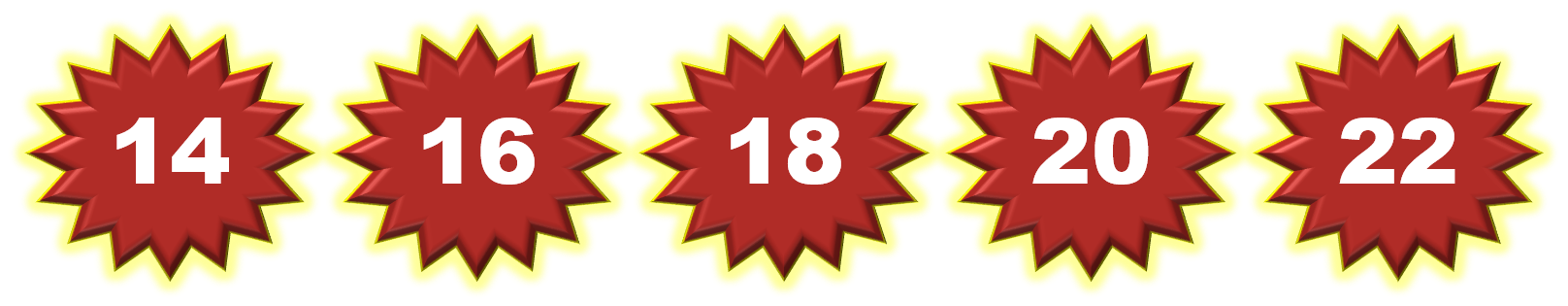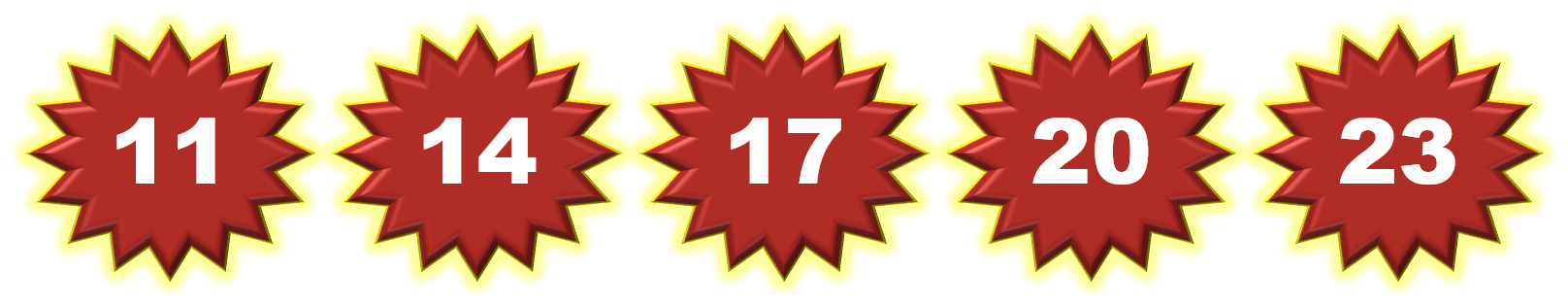1
visibility

Choose the correct counting pattern with the rule ‘add two’.

• A• BThe correct answer is Option A:Solution:
Considering the given options.

Option A.Option B.On Option A:  Considering the given number pattern.Here, the numbers are increasing.
So, we first find the rule.

Note that:
14 + 2 = 16
16 + 2 = 18
18 + 2 = 20
20 + 2 = 22

So, the rule is "add 2", and this is the required answer.

Hence, the correct counting pattern with the rule ‘add two’ is Option A: